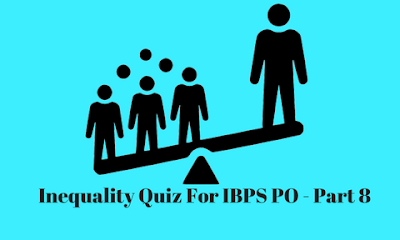# Inequality Quiz For IBPS PO - Part 8Directions (Q. 1-5): In the following questions, the symbols %, #, @, & and \$ are used with the following meaning as illustrated below:
• ‘P @ Q’ means ‘P is not greater than Q’.
• ‘P \$ Q’ means ‘P is neither greater than nor equal to Q’.
• ‘P % Q’ means ‘P is neither greater than nor smaller than Q’
• ‘P #Q’ means ‘P is neither smaller than nor equal to Q’
• ‘P & Q’ means ‘P is not smaller than Q’
Now, in each of the following questions assuming the given statements to be true, find which of the conclusions given below them is definitely true.

### Q1. Statements:

L @ K \$ M % N, K & O \$ J, P & M # Q
Conclusions: L @ N, O \$ M
1) Only I is true
2) Only II is true
3) Either I or II is true
4) Both I and II are true
5) Neither I nor II is true.
Ans. 2.
Solution:
L≤ K < M= N,   K ≥ O < J,   P ≥ M>Q

L≤ K < M = N,    O≤ K < M

### Q2. Statements:

L @ K \$ M % N, K & O \$ J, P & M # Q
Conclusions:L \$ M, O % N
1) Only I is true
2) Only II is true
3) Either I or II is true
4) Both I and II are true
5) Neither I nor II is true
Ans. 1.
Solution:
L≤ K < M= N,   K ≥ O < J,   P ≥ M>Q

L≤ K < M,    O≤ K < M= N

### Q3. Statements:

L @ K \$ M % N, K & O \$ J, P & M # Q
Conclusions: L @ P, L # Q
1) Only I is true
2) Only II is true
3) Either I or II is true
4) Both I and II are true
5) Neither I nor II is true.
Ans. 5.
Solution:
L ≤ K < M= N,   K ≥ O < J,   P ≥ M >Q

L ≤ K < M ≤ P,    L ≤ K < M > Q

### Q4. Statements:

L @ K \$ M % N, K & O \$ J, P & M # Q
Conclusions: P # N, P % N
1) Only I is true
2) Only II is true
3) Either I or II is true
4) Both I and II are true
5) Neither I nor II is true.
Ans. 3.
Solution:
L ≤ K < M= N,   K ≥ O < J,   P ≥ M >Q

P ≥ M = N

### Q5. Statements:

L @ K \$ M % N, K & O \$ J, P & M # Q
Conclusions: O \$ P, O \$ N
1) Only I is true
2) Only II is true
3) Either I or II is true
4) Both I and II are true
5) Neither I nor II is true.
Ans. 4.
Solution:
L ≤ K < M= N,   K ≥ O < J,   P ≥ M >Q

O ≤ K < M ≤ P,    O ≤ K < M= N#### What's trending in BankExamsToday

Smart Prep Kit for Banking Exams by Ramandeep Singh - Download here